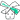# 学不会 Rxjs 是一件很丢脸的事情

📆September 21, 2016

## What Is RxJS

### Rx's Hello World

``````// auto-complete
const Observable = Rx.Observable
const input = document.querySelector('input')

const search\$ = Observable.fromEvent(input, 'input')
.map(e => e.target.value)
.filter(value => value.length >= 1)
.throttleTime(100)
.distinctUntilChanged()
.switchMap(term => Observable.fromPromise(wikiIt(term)))
.subscribe(
x => renderSearchResult(x),
err => console.error(err)
)``````

• 监听 `input` 元素的 `input` 事件
• 一旦发生，把事件对象 `e` 映射成 `input` 元素的值
• 接着过滤掉值长度小于 `1`
• 并且还设置了一个 `throttle` ( 节流器 )，两次输入间隔不超过 `100` 毫秒为有效输入
• 如果该值和过去最新的值相等的话，忽略他
• 最后，拿到值便调用 Wikipedia 的一个 API
• 最后的最后，需要 `subscribe` 才能拿到 API 返回的数据

## How It Works

### Observable

Rx 提供了一种叫 Observable 的数据类型，兼容 ECMAScript 的 Observable Spec Proposal 草案标准。他是 Rx 最核心的数据类型，结合了 Observer PatternIterator Pattern

Observable 其实就是一个异步的数组( ---> 2 minute introduction to rx )

``--a---b-c--d-----e--|-->  ``

`-` 表示时间轴，`a` ~ `e` 表示 emit 的值，`|` 则表示这个 stream 已经结束了。 比方说，`click` 事件用上图来表示：`a` 表示第 1 次点击，`b` 表示第 2 次点击，如此类推。

### Operators

``````const search\$ = Observable.fromEvent(input, 'input')
.map(e => e.target.value)
.filter(value => value.length >= 1)
.throttleTime(100)
.distinctUntilChanged()
.switchMap(term => Observable.fromPromise(wikiIt(term)))
.subscribe(
x => renderSearchResult(x),
err => console.error(err)
)``````

``````---i--i---i-i-----i---|--> (input)
map

---a--a---c-d-----c---|-->
b
filter

---a--a---c-d-----c---|-->
b
throttleTime

---a--a---c-------c---|-->
b
distinctUntilChanged

---a--a---c----------|-->
b
switchMap

---x--y---z----------|-->  ``````

## Learn By Doing

### Step 1

( 我要看 DEMO )

``````const canvas = document.querySelector('canvas')

const move\$ = Rx.Observable.fromEvent(canvas, 'mousemove')``````

``--m1---m1-m2--m3----m4---|-->  (mousemove)``

``````const move\$ = Rx.Observable.fromEvent(canvas, 'mousemove')
.map(e => ({ x: e.offsetX, y: e.offsetX }))``````

``````--m1---m2-m3--m4----m5---|-->  (mousemove)
map
--x1---x2-x3--x4----x5---|-->  (点坐标)``````

``````const move\$ = Rx.Observable.fromEvent(canvas, 'mousemove')
.map(e => ({ x: e.offsetX, y: e.offsetY }))
.bufferCount(2)``````

marble 图：

``````--m1---m2-m3--m4----m5---|-->  (mousemove)
map

--x1---x2-x3--x4----x5---|-->  (点坐标)
bufferCount(2)

-------x1-----x3----x5---|---> (两点坐标)
x2     x4``````

### Step 2

( 先让我要看看 DEMO )

``````const move\$ = Rx.Observable.fromEvent(canvas, 'mousemove')
.map(e => ({ x: e.offsetX, y: e.offsetY }))

const diff\$ = move\$
.zip(move\$.skip(1), (first, sec) => ([ first, sec ]))``````

``````--x1---x2-x3--x4----x5---|-->  (move\$)
skip(1)
-------x2-x3--x4----x5---|-->

--x1---x2-x3--x4----x5---|-->  (move\$)
-------x2-x3--x4----x5---|-->
zip

-------x1-x2--x3----x4---|-->  (diff\$)
x2 x3  x4    x5``````

### Step 3

( 我想看 DEMO )

``````-------x1-----x3----x5---|---> (bufferCount)
x2     x4

-------x1-x2--x3----x4---|-->  (diff\$)
x2 x3  x4    x5``````

`bufferCount` emit 的值依次为：`(x1, x2)``(x3, x4)` …… `x2``x3` 之间是有间隔的。这就是为什么线段会不连续的原因。

``````const move\$ = Rx.Observable.fromEvent(canvas, 'mousemove')
.map(e => ({ x: e.offsetX, y: e.offsetX }))
.bufferCount(2, 1)``````

``````--m1---m2-m3--m4----m5---|-->  (mousemove)
map

--x1---x2-x3--x4----x5---|-->  (点坐标)
bufferCount(2, 1)

-------x1-x2--x3----x4---|---> (两点坐标)
x2 x3  x4    x5``````

## Step 4

( 我就要看 DEMO )

``````const down\$ = Rx.Observable.fromEvent(canvas, 'mousedown')
const up\$ = Rx.Observable.fromEvent(canvas, 'mouseup')``````

``down\$.switchMapTo(move\$)``

``````--d---d-d-----d---d--|-->  (mousedown)
switchMapTo

--m---m-m-----m---m--|--> ``````

### Step 5

( 我只想看 DEMO )

`up\$``down\$` 组合成一个新的 stream ，但为了分辨他们，我们需要先把他们变换成新的 stream 。 查看文档 Combination Operators ---> mergeTransformation Operators ---> map

``````const down\$ = Rx.Observable.fromEvent(canvas, 'mousedown')
.map(() => 'down')
const up\$ = Rx.Observable.fromEvent(canvas, 'mouseup')
.map(() => 'up')

const upAndDown\$ = up\$.merge(down\$)``````

``````--d--d-d----d--d---|-->  (down\$)
----u---u-u------u-|-->  (up\$)
merge

--d-ud-du-u-d--d-u-|-->  (upAndDown\$)``````

``````upAndDown\$
.switchMap(action =>
action === 'down' ? move\$ : Rx.Observable.empty()
)``````

``````--d-ud-du-u-d--d-u-|-->  (upAndDown\$)
switchMap

--m-em-me-e-m--m-e-|-->``````

## Production

### Vue

``````new Vue({
el: '#app',
data: {
time: ''
},

created () {
this.timer\$ = Rx.Observable.interval(1000)
.map(() => new Date())
.map(d => moment(d).format('hh:mm:ss'))
.subscribe(t => {
this.time = t
})
},

beforeDestroy () {
this.timer\$.unsubscribe()
}
})``````

### Angular2

RxJS 已经是 Angular2 的标配，不多说。 更多可查看对应的文档 Angular2 - Server Communication

## You Might Not Need RxJS

• 多个复杂的异步或者事件组合在一起
• 处理多个数据序列（有一定顺序）